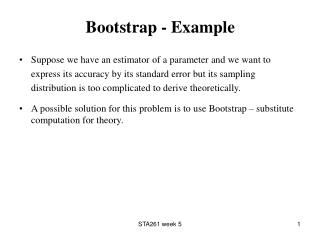DownloadDownload PresentationBootstrap - Example

# Bootstrap - Example

Télécharger la présentation## Bootstrap - Example

- - - - - - - - - - - - - - - - - - - - - - - - - - - E N D - - - - - - - - - - - - - - - - - - - - - - - - - - -
##### Presentation Transcript

1. Bootstrap - Example • Suppose we have an estimator of a parameter and we want to express its accuracy by its standard error but its sampling distribution is too complicated to derive theoretically. • A possible solution for this problem is to use Bootstrap – substitute computation for theory. STA261 week 5

2. Parametric Bootstrap • Suppose data are realization of a random variable with a probability distribution with density fθ(x) with θ unknown. • We begin the bootstrap process by first estimating θ from the data to get • Next we simulate B “bootstrap samples” from the density fθ(x) with θ being replaced by and for each bootstrap sample we calculate a “bootstrap estimate” of θ denoted by • Note that the bootstrap samples are always the same size as the original data set. • The bootstrap estimate of the s.e. of is the sample standard deviation of the bootstrap estimates STA261 week 5

3. Example • Consider a data set containing breakdown times of an isolative fluid between electrodes. • The theoretical model for this data assumes that this is an i.i.d sample from an exponential distribution… • The method of moment estimator of λ is…. • We want the s.e of this estimator and for this we use parametric bootstrap. STA261 week 5

4. Empirical Distribution • The empirical distribution is the estimate for the probability distribution that generated the data. • The observed data are the possible values and are equally likely. • The empirical distribution assign a probability of 1/n to each data value. STA261 week 5

5. Nonparametric Bootstrap • If we could take an infinite number of samples of size n from the probability distribution that generated the data and for each sample find , we would know the sampling distribution of . • In the nonparametric bootstrap procedure we get bootstrap samples of size n by re-sampling from the data. • Re-sampling is sampling with replacement from this empirical distribution. STA261 week 5

6. Parametric Versus Nonparametric Bootstrap • In the parametric bootstrap we have to make an assumption about the form of the distribution that generated the data • Non-parametric – if n is small can behave oddly. STA261 week 5